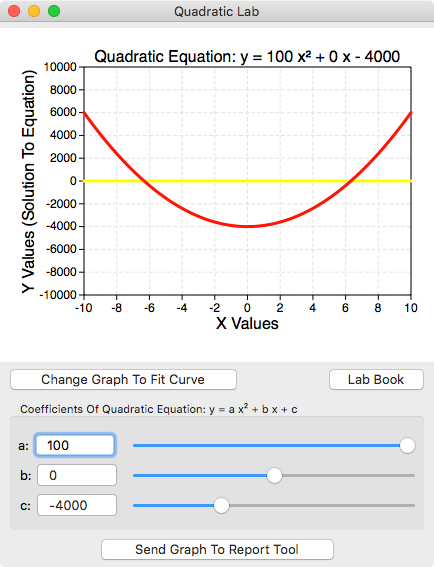Quadratic Lab shows what happens when you change the values of a, b and c in the quadratic equation:

y = a x2 + b x + c

a, b and c are called coefficients. You can change those coefficients using the slider controls. The following shows a picture of Quadratic Lab.The yellow line shows the roots of the quadratic equation. If there is no yellow line then there are not roots. See the Lab Book provided on the Quadratic Lab interface for additional information.